/

### Standard Statistical Tests for Normality: The Anderson-Darling test

The Anderson-Darling test tests the null hypothesis that a sample,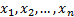comes from a pre-specified population distribution (or a pre-specified family of such distributions).

In its basic form, the test assumes that there are no parameters to be estimated for the distribution being tested, in which case the test and its set of critical values are distribution-free.

However, it is most commonly used where a family of distributions are being tested. For example, we might be testing whether the sample comes from a Normal distribution but without specifying in advance the mean and standard deviation of that distribution. It then becomes necessary to estimate the parameters on which the particular distribution depends and this needs to be taken into account by adjusting the test statistic and/or its critical values.

The test was published in 1952, see Anderson and Darling (1952). It is based on the observation that if the data does come from the hypothesised distribution then the data can be transformed to what should be a uniform distribution. The transformed data can then be tested for uniformity with a distance test, see e.g. Shapiro (1980).

In its basic form, it involves the following test statistic,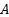, where we are testing the null hypothesis that the data is coming from a distribution with cumulative distribution function (cdf)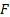: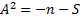where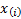is the’th order statistic, i.e. the’th smallest value in the sample and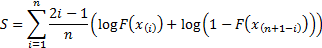Essentially the same approach can be used when testing whether data comes from a pre-specified family of distributions. However, the statistic must then be compared against critical values appropriate to the family in question and dependent also on the method used for parameter estimation.

A ‘K-sample’ Anderson-Darling test can be used to test whether several samples appear to be coming from a single distribution, without the need to specify in advance what the distributional form might be. Sholz and Stephens (1987) indicate how this basic approach can be used to test whether a number of random samples with possibly different sample sizes are coming from the same underlying distribution, where this distribution is unspecified in advance.